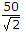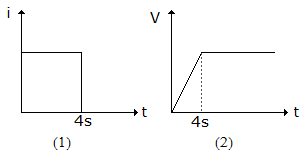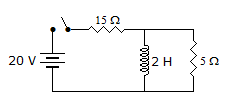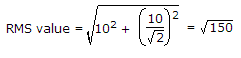# Electronics and Communication Engineering - Networks Analysis and Synthesis

46.

A sinusoidal voltage has peak to peak value of 100 V. The rms value is

 A. 50 V B. 70.7 V C. 35.35 V D. 141.41 V

Explanation:

Peak value = 50 V, rms value == 35.35 V.

47.

A capacitor is needed for an ac circuit of 230 V, 50 Hz the peak voltage rating of the capacitor should be

 A. 230 V B. 0.5 x 230 V C. 2 x 230V D. 230/2V

Explanation:

Peak voltage rating = 2 (rms voltage rating).

48.

The current wave shape shown in figure (1) is applied to a circuit element. The voltage across the element is shown in figure (2). The element isA. R B. L C. C D. Semiconductor

Explanation:

When a current pulse is applied to a capacitor the voltage rises linearly and becomes constant at the end of pulse.

49.

In figure, the current supplied by battery immediately after switching on the circuit isA. 1 A B. 4 A C. 10 A D. 0

Explanation:

At t = 0, inductance behaves as open circuit.

50.

RMS value of i(t) = 10[1 + sin (- t)] is

 A. 10 B. 10/2 C. l50 D. 102.